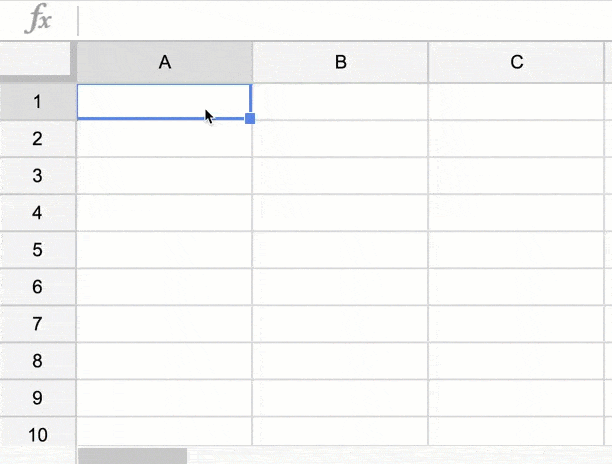# Google Sheets: Construct Formulas with the INDIRECT Function

One of many things that is great about spreadsheets is the ability to use formulas to create patterns. This can oftentimes be a huge timesaver from manually typing a long list. For example, if I have first names in one column and last names in another column I can write a formula to put them into the format of last, first.## Construct Using INDIRECT

When you need to construct a formula instead of directly type a formula, use the INDIRECT function. It turns text strings into a cell reference. For example, if you need to have a formula that references a column of data. You may want the same formula for a different column.

For example, if you have lists of data in different columns on Sheet1 and want to bring up a particular column. =Sheet1!A1 would return the value in cell A1 from Sheet1. Dragging the formula would result in the cell reference adjusting.To adjust for a different column, construct a formula that references the desired column. In the Formula =Sheet1!A1 the reference to column A would need to change to column B, C, etc… Using INDIRECT allows you to utilize cell referencing and concatenation to build the desired formula. If in cell B1 you were to identify the column you wish to reference you would need to concatenate the text string of Sheet1! with cell B1 and the row reference. =INDIRECT(“Sheet1!”&B1&”1”) would join the text string of the sheet name with the cell that contains the column value and the row value.## Indirect Webinar

Christine Pinto and I went through a use case of the INDIRECT function in the webinar below.

## Subscribe to the Alice Keeler Newsletter